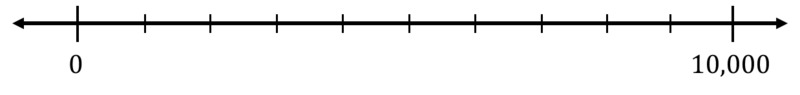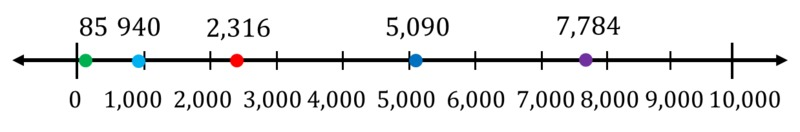# Rounding to the Nearest 1000

Alignments to Content Standards: 4.NBT.A.3

The tick marks on the number line are evenly spaced. Label them.Plot the following numbers on the number line:

85

940

2,316

5,090

7,784

Round each number to the nearest 1000. Explain how you can tell which thousand each number will round to by looking at the number line.

## IM Commentary

The purpose of this task is for students to estimate the position of numbers less than 10,000 on the number line, to practice rounding, and to make the connection between rounding and location on the number line. This task naturally builds off of 3.NBT, 4.NBT Rounding to the Nearest 100 and 1000, which in turn builds off of 3.NBT Rounding to the Nearest Ten and Hundred.

## Solution

The numbers are all plotted on the number line below with the small tick marks labelled:For rounding to the nearest 1000 we first find which two thousands the number lies between and round to the nearer of the two.

85 lies between 0 and 1000. 500 is exactly half way between 0 and 1000, and 85 is between 0 and 500. So 85 is closer to 0 than 1000 on the number line and it rounds down to 0.

940 lies between 0 and 1000. 500 is exactly half way between 0 and 1000, and 940 is between 500 and 1000. So 940 is closer to 1000 than 0 on the number line and it rounds up to 1000.

2,316 lies between 2,000 and 3,000. 2,500 is exactly half way between 2,000 and 3,000, and 2,316 is between 2,000 and 2,500. So 2,316 is closer to 2,000 than 3,000 on the number line and it rounds down to 2,000.

5,090 lies between 5,000 and 6,000. 5,500 is exactly half way between 5,000 and 6,000, and 5,090 is between 5,000 and 5,500. So 5,090 is closer to 5,000 than 6,000 on the number line and it rounds down to 5,000.

7,784 lies between 7,000 and 8,000. 7,500 is exactly half way between 7,000 and 8,000, and 7,784 is between 7,500 and 8,000. So 7,784 is closer to 8,000 than 7,000 on the number line and it rounds up to 8,000.